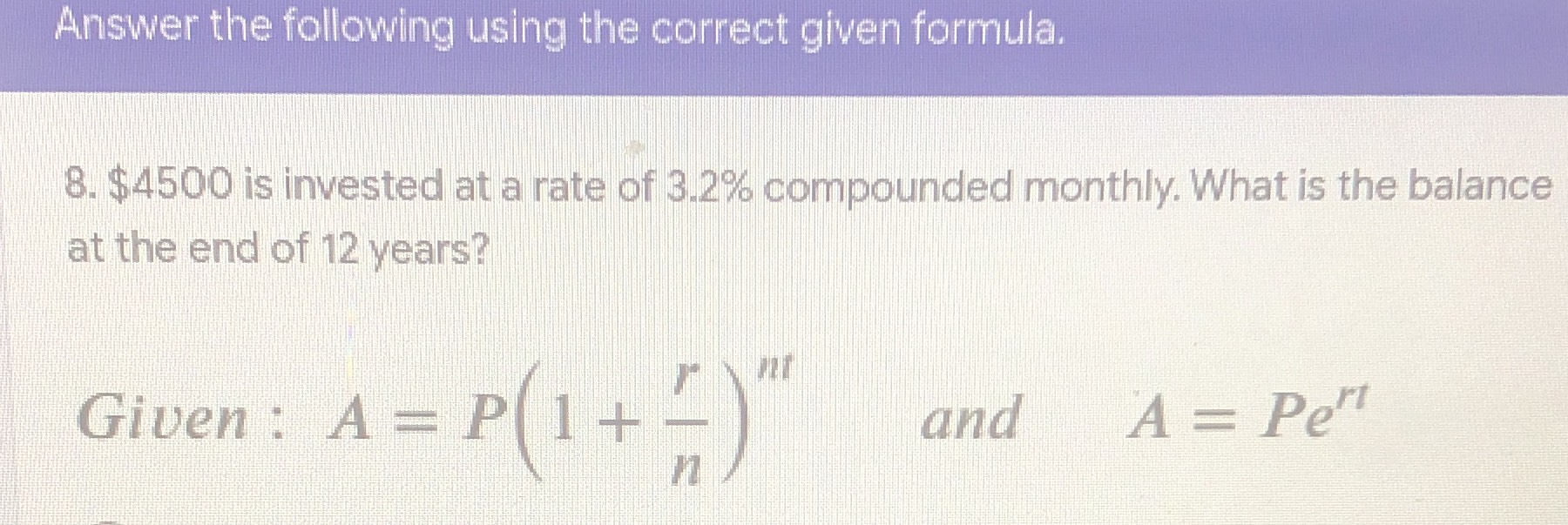### ¿Todavía tienes preguntas de matemáticas?

Pregunte a nuestros tutores expertos
Algebra
Pregunta$$8 . \ 4500$$ is invested at a rate of $$3.2 \%$$ compounded monthly. What is the balance at the end of $$12$$ years? Given : $$A = P ( 1 + \frac { r } { n } ) ^ { n t }$$ and $$A = P e ^ { r t }$$

$$419845$$